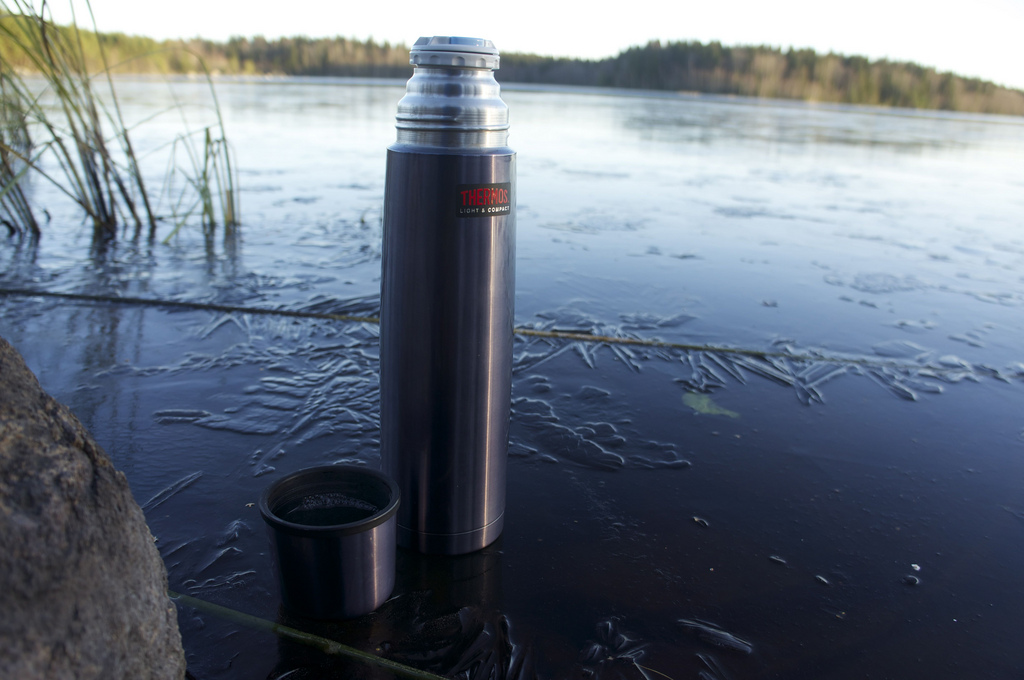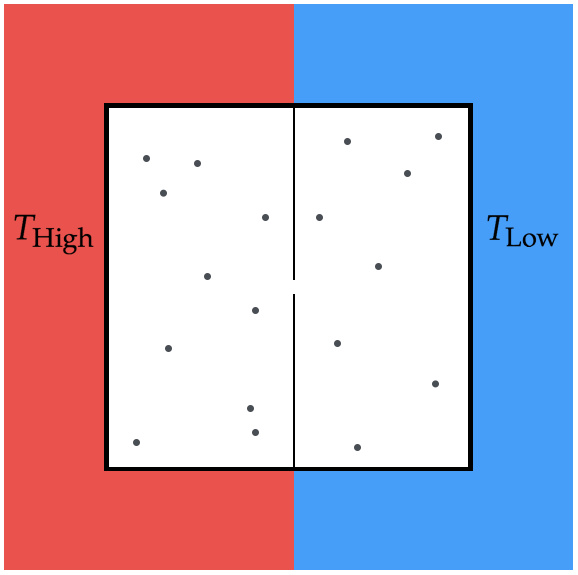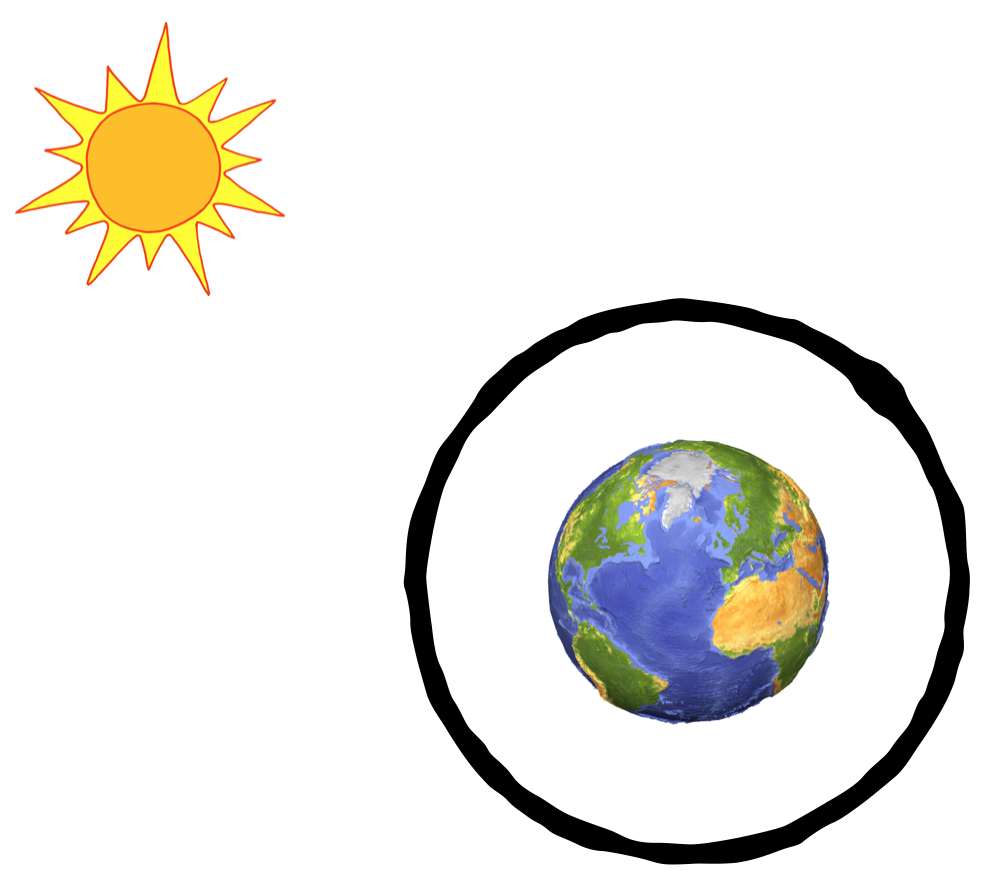Classical Mechanics

# Statistical Thermodynamics: Level 3-5 ChallengesOver the course of a day, the outside temperature varies in a periodic fashion that can be well approximated by a sinusoid. Suppose you leave a thermos of coffee outside in the shade, measuring its temperature throughout the day, and find that it varies from 70 to 80 deg F.

Now, you make coffee in the same thermos at 200 deg F and leave it on the desk in your room. About how long (in hours) does it take for the coffee to cool to 140 deg F?

Assumptions and Details

• The outside temperature varies with time according to $T(t) = \left(75+ 15 \cos \omega t\right)\ \text{deg F}$ where $\omega = 2\pi / 24\ \text{hr}^{-1}$.
• Your room is 75 deg F.
• Your coffee follows Newton's law of cooling: $\partial_t T_\text{cup} \propto \left(T_\text{env} - T_\text{cup}\right)$.Consider a gas in the device shown above. Two halves of the box are separated by a dividing wall that has a small hole in it, which is just slightly wider than the diameter of the gas molecules. Each half of the box is in contact with a heat bath held at a given temperature.

If there are $N$ gas molecules in the box, find the expected number of gas molecules in the hot side when the box reaches steady state.

Assumptions and Details

• $N=100,000$
• $T_\text{High}=700\text{ K}$
• $T_\text{Low}=200\text{ K}$Earth is surrounded by a layer of atmosphere that is transparent to solar radiation (i.e. the atmosphere material cannot be heated directly by the Sun), but can retain and radiate infrared heat from the Earth (which can be heated by the Sun).

Suppose an enormous amount of black soot enters the atmosphere (perhaps from volcanos or nuclear weapons) that can be heated by the Sun directly. When the soot surrounded Earth reaches equilibrium, it has the stable temperature $T_{soot}$.

Find $T_{soot}/T_0$, where $T_0$ is the temperature of the Earth with no atmosphere whatsoever.

Assumptions

• The surface of the Earth and the soot layer are perfect blackbodies.
• Approximate the soot as a spherical shell around the Earth.Tesla decides to go back to basics with their new line, the Entropica series. Leaving aside their rather successful venture into electric engines, Tesla pivots to embrace the heat engine, which uses two heat blocks of initial temperature $T_+$, and $T_-$ to drive an engine through a thermodynamic cycle (pictured above).

Concretely, each cycle drives some heat $\delta Q$ from the hot reservoir to the cold reservoir, and the engine performs some work $\delta W$ to propel the car, such that the engine returns to its original state after each cycle. Clearly, each cycle lowers the temperature $T_+$, and raises the temperature $T_-$, until $T_+=T_-=T^*$, at which point the engine can do no further work.

Find $T^*$ (in deg Kelvin) at the point where the engine stops running.

Assumptions and Details

• The entire process is thermodynamically reversible.
• $T_+$ = 423$^\circ$ K
• $T_-$ = 300$^\circ$ K
• Both heat reservoirs are metal blocks of heat capacity $\gamma$Three objects of same heat capacity with temperature $T_1=200~\mbox{K}$, $T_2=400~\mbox{K}$ and $T_3=400~\mbox{K}$ exchange heat with each other. They are isolated from the rest of the universe. Find the highest possible temperature one of them can reach in kelvin.

Hint: The first and second laws of thermodynamics are your friend.

×